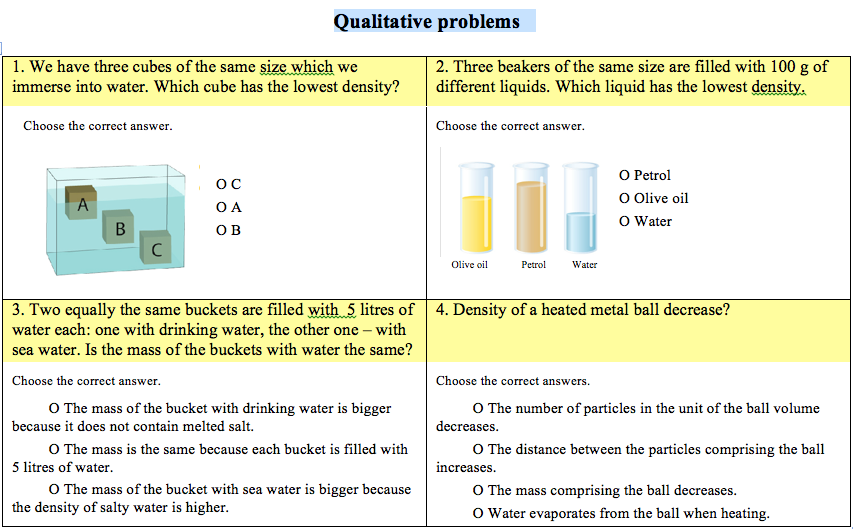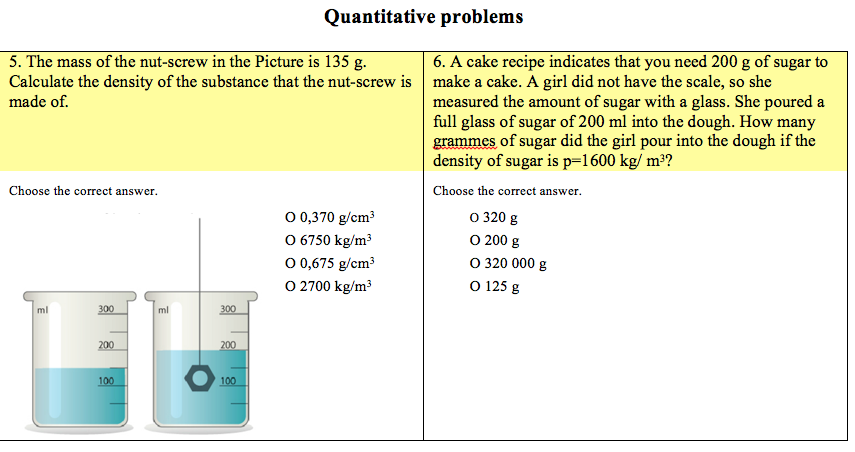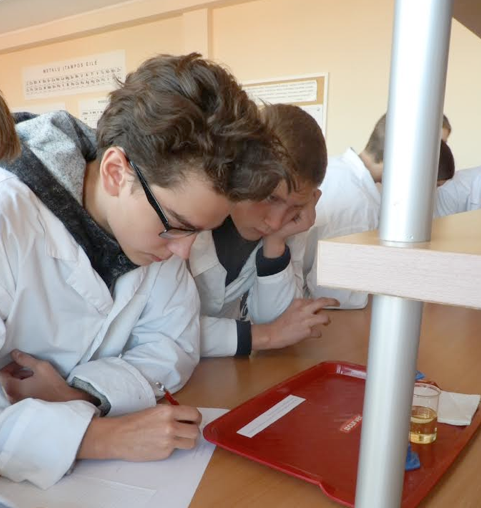Physics
Chem
Substance density
Author
Birute Rakauskiene and Lina kankeviciene; Alytaus Kolegija
Target Group
Brief Description
Solving problems using the density formula. Integrated lesson of Physics and Chemistry for 8th grade

Share

# Substance density

### Solving problems using the density formula. Integrated lesson of Physics and Chemistry for 8th grade

Type of the lesson: Problem solving.

Subject of the lesson: Substance density.

Goal: Learn to solve experimental problems of various complexity using the density formula.

Objectives of the lesson: In pairs and small groups, using the tables of substance density, formulae, substances and materials, you will do the following:
1. Solve experimental problems of complexity;
2. Check the results and assess yourselves.

Methods:
Group work (pupils are divided into groups considering the number of pupils in the class),
Practical experiment (a group of pupils perform an experiment described in the task sheet)
Discussing specific situations, generalization and submission of the conclusions (in groups, pupils fill in the task sheets),
Presentation of the work to the class (one or two pupils from the group present the work to the class),
Self-assessment (pupils assess the knowledge gained according to the number of correct answers).

Procedure of the lesson:
At the beginning of the lesson, the subject and objectives of the lesson are announced. Pupils make groups for work (they may be divided into groups by the teacher) (5-7 min.)

At the beginning of the lesson a few qualitative (1-4) and quantitative (5, 6) problems are provided in slides.

Pupils work in groups according to the tasks given. (Teachers of Physics and Chemistry monitor the pupils’ work, advise them when needed on how to proceed with the work (12-15 min.).

Pupils’ groups present the tasks performed to the whole class (The screen shows the task or task answers if possible). Pupils of the class correct the mistakes noticed, evaluate and discuss). (12-15 min.).

Pupils perform tasks 11 and 12 of the workbook (handouts  may be given). Correct answers of the task are provided. (Pupils assess themselves according to the number of correct answers) (5-7 min.).

Generalization of the lesson. Teachers assess pupils’ work (5 min.)